# Korovkin theorems

(diff) ← Older revision | Latest revision (diff) | Newer revision → (diff)

Korovkin's first theorem states that if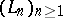is an arbitrary sequence of positive linear operators on the spaceof real-valued continuous functions on the interval(cf. Continuous functions, space of; Linear operator) and iffor all, where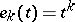(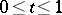,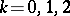), thenfor all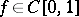.

The statement of Korovkin's second theorem is similar to that of the first theorem, but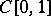is replaced by(the space of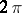-periodic real-valued functions on, endowed with the topology of uniform convergence on) andis taken from the set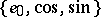.

These theorems were proved by P.P. Korovkin in 1953 ([a3], [a4]). In 1952, H. Bohman [a2] had proved a result similar to Korovkin's first theorem but concerning sequences of positive linear operators onof the form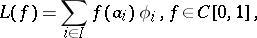where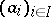is a finite set of numbers in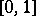and(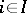). Therefore Korovkin's first theorem is also known as the Bohman–Korovkin theorem. However, T. Popoviciu [a5] had already proved the essence of the theorem in 1950.

Korovkin has tried to generalize his first theorem by replacing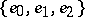with other finite subsets of. He has shown that if a subset"behaves like", then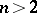(Korovkin's third theorem). Moreover, he showed that a subset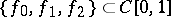"behaves like"if and only if it is a Chebyshev system of order two.

The Korovkin theorems are simple yet powerful tools for deciding whether a given sequence of positive linear operators onor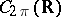is an approximation process. Furthermore, they have been the source of a considerable amount of research in several other fields of mathematics (cf. Korovkin-type approximation theory).

See [a1] for a modern and comprehensive exposition of these results and for (some) applications.

How to Cite This Entry:
Korovkin theorems. Encyclopedia of Mathematics. URL: http://encyclopediaofmath.org/index.php?title=Korovkin_theorems&oldid=13016
This article was adapted from an original article by F. Altomare (originator), which appeared in Encyclopedia of Mathematics - ISBN 1402006098. See original article